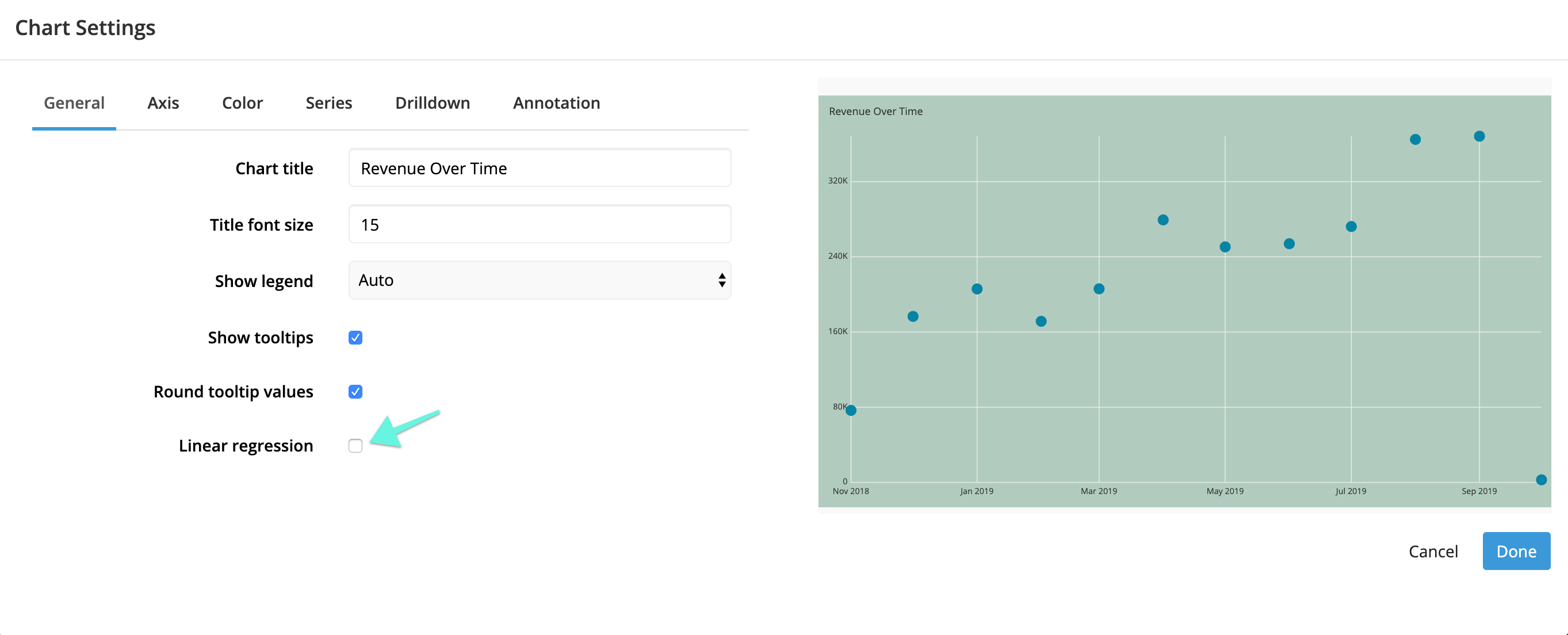# Linear regression line in Scatter Plot charts

Charts

It’s often handy to add trend lines to your scatter plots to quickly see the general trends occurring in your data.

An option in the settings will allow you to add a linear regression line to your Scatter Plot. A linear regression line enables you to view the trend line of your Scatter Plot dataset at a glance. Adding one in Chartio is as simple as checking a checkbox in your chart settings.

Chartio uses a best-fit algorithm with equal weighting on all data points to generate the regression line. We use the least-squares criterion to estimate the regression line. The regression line is sometimes called the “line of best fit” because it is the line that fits best when drawn through the points. It is a line that minimizes the distance of the actual scores from the predicted scores.

A line of best fit (or “trend” line) is a straight line that best represents the data on a Scatter Plot. This line may pass through some of the points, none of the points, or all of the points.In the Settings, under the General tab, check the Linear Regression checkbox. Click “Done” to preview your changes in the Data Explorer.Be sure to save your changes before exiting the Data Explorer.

As a final note, your trend line will use all the data present on your chart for the calculation. If you would like to remove some outliers from the trend line calculation, you will need to filter those data from the chart as well. It is not possible to just omit them from the regression line calculation.

See an example of adding a trend line to your Scatter Plot in the short video below:

See more